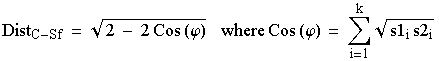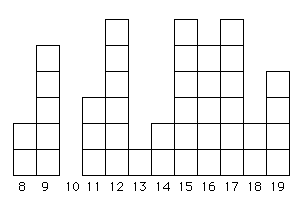Lecture 6. Population Genetics IV: Genetic distances -- biological vs. geometric approaches.

Go to web page outlining major aspects of analyzing genetic population structure (WAAP.html)
(some important measures to calculate, very basic intro. to the practicalities of running a few of the many software choices)

Taxonomy of genetic distance measures.

We began our study of population genetics by developing the concept of hetero- and homozygosity from Hardy-Weinberg principles. We used a Hardy-Weinberg approach as one way to get at a measure of subpopulation differentiation in terms of F-statistics. The F-statistics provide a view of the variance structure of populations, and can provide an overall comparison of the degree to which populations are structured
FST = 0 meaning no structure, no differentiation, and
F
ST = 1 meaning completely differentiated;
F
IS = 0 meaning neither inbreeding nor outbreeding (i.e., meeting the random mating Hardy-Weinberg expectation),
F
IS = 1 meaning completely inbred,
F
IS = -1 meaning completely outbred.

Go to web page describing how to calculate FST from heterozygosities (FST.html)

F-statistics do not, however, easily allow pairwise comparisons among subpopulations or populations. That is, we can assess pairwise FST between populations, but those pairwise "distances" take account only of the data for the two populations concerned, not all the data simultaneously. We would like a way to quantify the degree to which A differs from B, B from C, and A from C from the entire pool of data. We can do this in two major ways -- with or without underlying biological models. The latter (no biological assumptions or model) are also known as geometric distances. These geometric distances include Rogers and Cavalli-Sforza chord distances. Distance measures that do make biological assumptions include Reynolds and Neis distances. Lets examine each in turn.

1) Distance methods with no biological assumptions. A locus-specific, codominant marker population genetic data set, such as the bear one you have used for homeworks, consists of a set of individual- and population-indexed gene frequencies at one or more loci. We can analyze these data as a set of numbers without making any biological assumptions. Approaches could include principal components analyses (PCA), Euclidean distances or somewhat more complex geometric distances.  Many of these will allow us to create a sort of abstract "map" of the populations in one, two, three or more dimensions (obviously, maps with dimensionalities > 3 are hard to visualize). Some of these maps can be condensed into matrices of distances. Heres an example using real microsatellite data for Western Scrub-Jays (Aphelocoma californica).
Table 6.1.  Cavalli-Sforza chord distances for five populations of Western Scrub-Jays, Aphelocoma californica.

 WOb3 WSp3 WCal WOoc WSp2 WOb3 0 0.0332 0.0492 0.0428 0.0466 WSp3 0.0332 0 0.0488 0.0645 0.0449 WCal 0.0492 0.0488 0 0.0617 0.0533 WOoc 0.0428 0.0645 0.0617 0 0.058 WSp2 0.0466 0.0449 0.0533 0.058 0
The table entries are Cavalli-Sforza chord distances (Cavalli-Sforza and Edwards, 1967; described on pp. 163-166 of Weir, 1996) between five jay populations. For example, the "distance" between population WSp3 and population WOb3 is 0.0332, which is smaller than the distance of 0.0488 between WSp3 and WCal. The matrix is symmetrical (A to B = B to A) and has zeros on the diagonal (A to A = 0).

How did we get these Cavalli-Sforza distances? They are simply a geometric view of the distances between multi-dimensional points on a hypersphere (a sphere with > 3 dimensions). Say we have two subpopulations S1 and S2 assayed at a single locus with alleles i = 1 to k. The formal definition is:Eqn 6.1

That is, we take the square root of the frequency of allele 1 in S1 times that of allele 1 in S2 and repeat and sum that quantity for all k alleles. That gives us Cos (y) which we can plug into the square-root term on the RHS (right hand side) of Eqn 5.1 above. I dont expect you to use or memorize this -- just to see that it is a purely numerical/geometric approach. If we were doing it in 3 dimensions it would be akin to figuring out the distance from New York to London along the surface of the globe (called the chord distance). It can be fairly easily incorporated into a number-crunching computer program that will produce output like the table of Cavalli-Sforza distances shown above. Those distances, in matrix form, can then be used as input for phylogenetic tree-building routines such as the UPGMA, Fitch-Margoliash and neighbor-joining approaches we used in the homeworks.

The Cavalli-Sforza chord distance was an early measure and is still used (in fact I see it gaining ground for use with microsatellites). Another geometric distance that was widely used with allozymes (but I have not seen used with microsatellite data) is Rogers distance (Wright, 1978). One reason the Cavalli-Sforza distance may be in greater current use is that it was specifically evaluated (and performed well) in simulations of tree-building algorithms by Takezaki and Nei (1996). [For all we know Rogers distance may perform equally well or better under circumstances that would apply well to the questions people like me seek to address -- but since no one has done such a study, people like me will tend to go with one that has a documented good track record]. A very important part of the robustness of a distance measure is its performance under a variety of conditions. It is always best if we can compare several distance measures under conditions in which we know what the answer should be. Paetkau et al. (1997) provide an evaluation of various distance measures that apply to distance measures potentially useful for microsatellite analysis of bear populations.

2) Distance methods with biological assumptions. With a little luck (or a lot of hard work), we know something about the evolutionary forces (most importantly here mutation and drift, since we assume we are using markers that are not subject to natural selection) driving genetic change in the system we're interested in. If so, it seems reasonable to take advantage of that knowledge by incorporating it into building a distance model. After all, we expect models with greater realism to perform better (albeit at the cost of greater complexity, usually). Several distance measures incorporate assumptions about the importance of drift and mutation as forces of change:

Reynolds distance or the "coancestry" distance (Reynolds et al., 1983; see Weir, 1996, p. 167)
Neis distance (Nei 1972, 1978)
Models using a stepwise mutation model (SMM) specifically developed for microsatellites (e.g., dm2[delta mu squared] of Goldstein et al., 1995).

The problem with making assumptions is that violations can cause errors. Empirically, it appears that many of the stepwise mutation models for microsatellites do not perform well when analyzing many (most?) data sets, especially those where small population sizes mean that drift has played at least as large a role as mutation. Reynolds distance, which was derived for allozyme data on small (e.g., vertebrate) populations assumes a primary role for drift and is an infinite-alleles model (an allele can change from any given state into any other given state). Reynolds reliance on "drift only" seemed inappropriate for microsatellites, which have:
a) a mutation rate that appeared clearly much larger than that of allozymes (1 mutation per 1,000 or 10,000 replication events for microsatellites vs. 1 mutation per 1,000,000 replication events for allozymes). [But that may be based on very long repeats in highly polymorphic human populations].
b) a mutation process that would seemingly not fit the infinite-alleles model because mutations generally occur in "stepwise" fashion by adding or deleting one of a series of beads (AC10 goes to AC9 or AC11, where the subscript refers to the number of AC repeat units).
[See my web page http://www.uwyo.edu/zoology/mcdonald/dna.htm for a quick overview of microsatellites].
Nevertheless, Reynolds' distance and its neglect of the importance of mutation,  may work better than we would have expected (at least in some species/populations) for two reasons:
a) small population sizes (= high potential for drift)
b) "missing steps" because drift creates a "chunky" distribution of alleles instead of the smooth bell curve we would expect under a strict stepwise process.Fig. 6.1. A microsatellite allele frequency distribution under a strict stepwise mutation model (SMM). The X-axis shows the number of repeat units (e.g., AC8 to AC19), while the Y-axis shows the number of alleles. Starting with either a 13 or 14 repeat chain as the ancestor, we tend to accumulate more alleles at sizes close to the starting point because of equal likelihood of additions or subtractions and because larger changes (a variant of "mutations of large effect") will tend to be rare (we think).Fig. 6.2. An allele frequency distribution that has been greatly affected by drift and may better fit an infinite-alleles model (IAM). Even if the mutations that generated the original variation did occur in stepwise fashion, drift has removed some allele sizes (e.g., the 10-repeat category) while randomly selecting others to be greatly over-represented (e.g., 12, 15 and 17). This sort of "chunky" distribution may be quite common in many natural populations of vertebrates (where effective population sizes, Ne, are always small or at least often fluctuate to low numbers).

Infinite-alleles vs. stepwise mutation models: Infinite-alleles models were the standard approach for most allozyme analyses because it was difficult or impossible to predict the state of a mutation from knowledge of the state of its ancestors. That is, given that we had one allelomorph (protein/enzyme of a given size/charge that showed a particular electrophoretic banding profile) it was not at all clear how it related to other allelomorphs. In a stepwise model, in contrast, one assumes that an allele of a given size has as its ancestor either the allele one repeat unit longer or the allele one repeat unit shorter.
Equations for genetic distances:
Download a pdf of equations for various measures of genetic distance.  This pdf was once on the NC State website (statistical genetics home base for Weir and Cockerham).
http://statgen.ncsu.edu/brcwebsite/software_BRC.php
{The NC site has several software programs and a link to many others at: http://www.nslij-genetics.org/soft/}
References: Cavalli-Sforza, L.L. and A.W.F. Edwards. 1967. Phylogenetic analysis: models and estimation procedures. Am. J. Hum. Gen. 19: 233-257.

Goldstein, D. B., A.R. Linares, L.L. Cavalli-Sforza, and M.W. Feldman. 1995. Genetic absolute dating based on microsatellites and the origin of modern humans. PNAS USA 92: 6723-6727.

Nei, M. 1972. Genetic distance between populations. Am. Nat. 106: 283-292.

Nei, M. 1978. Estimation of average heterozygosity and genetic distance from a small number of individuals. Genetics 76: 379-390.

Paetkau, D., L.P. Waits, P.L. Clarkson, L. Craighead, and C. Strobeck. 1997. An empirical evaluation of genetic distance statistics using microsatellite data from bear (Ursidae) populations. Genetics 147:1943-1957.

Reynolds, J., B.S. Weir, and C.C. Cockerham. 1983. Estimation of the coancestry coefficient: Basis for a short-term genetic distance. Genetics 105: 767-779.

Takezaki, N., and M. Nei. 1996. Genetic distances and reconstruction of phylogenetic trees from microsatellite DNA. Genetics 144: 389-399.

Weir, Bruce S. 1996. Genetic Data Analysis II: Methods for discrete population genetic data (2nd. ed.). Sinauer Assoc., Sunderland, MA.

Wright, S. 1978. Evolution and the Genetics of Populations, Vol. 4: Variability Within and Among Natural Populations. University of Chicago Press, Chicago.